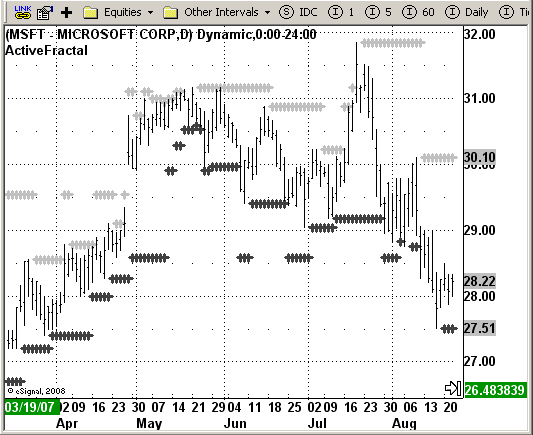# Bill Williams ActiveFractal

ICE Data Services -

ABillW_ActiveFractal.efs                                                                    EFSLibrary - Discussion Board

File Name: ABillW_ActiveFractal.efs

Description:
Bill Williams. ActiveFractal

Formula Parameters:

Notes:
According to Bill Williams, one should enter the market after the new
price top (for long positions) or bottom (for short positions) has
been broken through. The identification here is based on defining the
genuine fractal formation; an upward fractal is detected if there is a
row (at least three) increasing values and after the local top has been achieved,
bars (at least two bars) show a constant descent. So the model requires at least
5 bars of which the middle ont is the local top (upward fractal) or the local
bottom (downward fractal)

This indicator highlights periods suited for buying in Light Grey, an periods
suited for selling in Dark Grey.

ABillW_ActiveFractal.efsEFS Code:

```/*********************************
Provided By:
eSignal (Copyright c eSignal), a division of Interactive Data
Formula Script (EFS) is for educational purposes only and may be
modified and saved under a new file name.  eSignal is not responsible
for the functionality once modified.  eSignal reserves the right
to modify and overwrite this EFS file with each new release.

Description:
Bill Williams. ActiveFractal

Version:            1.0  10/14/2008

Notes:
According to Bill Williams, one should enter the market after the new
price top (for long positions) or bottom (for short positions) has
been broken through. The identification here is based on defining the
genuine fractal formation; an upward fractal is detected if there is a
row (at least three) increasing values and after the local top has been achieved,
bars (at least two bars) show a constant descent. So the model requires at least
5 bars of which the middle ont is the local top (upward fractal) or the local
bottom (downward fractal)

This indicator highlights periods suited for buying in Light Grey, an periods
suited for selling in Dark Grey.

Formula Parameters:                     Default:

**********************************/

function preMain()
{
setPriceStudy(true);

setStudyTitle("ActiveFractal");

setCursorLabelName("upFract", 0);
setCursorLabelName("dnFract", 1);

setDefaultBarFgColor(Color.darkgrey, 1);
setDefaultBarFgColor(Color.lightgrey, 0);

setPlotType(PLOTTYPE_DOT,0);
setPlotType(PLOTTYPE_DOT,1);

setDefaultBarThickness(3,0);
setDefaultBarThickness(3,1);
}

var bInit = false;
var xma5 = null;
var xma8 = null;
var xma13 = null;
var xHH = null;
var xLL = null;

function main() {

if ( bInit == false ) {
xma5 = sma(5, hl2());
xma8 = sma(8, hl2());
xma13 = sma(13, hl2());
xHH = upperDonchian(2);
xLL = lowerDonchian(2);
bInit = true;
}

if (getCurrentBarCount() < 13) return;

var Value1 = xHH.getValue(0);
var Value2 = xLL.getValue(0);

var Price = 0;
var blBlue = xma13.getValue(-8);
var blRed = xma8.getValue(-5);
var blGreen = xma5.getValue(-3);
var stp_now = false;
var i1 = 1;
var maxFracsBack = 10;
var SwingLow = 0;
var SwingHigh = 0;
var J = 2;
var Found = false;
var Counter = 0;
var X = 0;
var Truth = false;
var dnFrac = 0.0;
var upFrac = 0.0;

for (i1 = 1; (i1 < maxFracsBack) && (SwingLow != -1) && (stp_now == false); i1++)
{
J = 2;
Found = false;
Counter = 0;
for (J = 2;(J < 80)&&(Found == false); J++)
{
Price = low(-J);
X = J + 1;
Truth = true;
for (X = (J + 1); ((X - J) <= 2)&&(Truth); X++)
{
if (Price > low(-X)) Truth = false;
}
X = J - 1;
for (X = (J - 1);((J - X) <= 2)&&(Truth); X--)
{
if (Price >= low(-X)) Truth = false;
}
if (Truth) Counter++;
if (Counter >= i1) Found = true;
}
if (Found) SwingLow = Price;
else SwingLow = -1;
if (((SwingLow > blRed)||(SwingLow > Value2)) == false) stp_now = true;
}
if (stp_now) dnFrac = SwingLow;
else dnFrac = -1;
stp_now = false;
i1 = 1;
for (i1 = 1;(i1 < maxFracsBack)&&(SwingHigh != -1)&&(stp_now == false);i1++)
{
J = 2;
Found = false;
Counter = 0;
for (J = 2;(J < 80)&&(Found == false); J++)
{
Price = high(-J);
X = J + 1;
Truth = true;
for (X = (J + 1); ((X - J) <= 2)&&(Truth); X++)
{
if (Price < high(-X)) Truth = false;
}
X = J - 1;
for (X = (J - 1);((J - X) <= 2)&&(Truth); X--)
{
if (Price <= high(-X)) Truth = false;
}
if (Truth) Counter++;
if (Counter >= i1) Found = true;
}
if (Found) SwingHigh = Price;
else SwingHigh = -1;
if (((SwingHigh < blRed)||(SwingHigh < Value1)) == false) stp_now = true;
}
if (stp_now) upFrac = SwingHigh;
else upFrac = -1;
var Res1 = null;
var Res2 = null;
if ((upFrac != 0)&&(upFrac != -1))
{
Res1 = upFrac;
}
if ((dnFrac != 0)&&(dnFrac != -1))
{
Res2 = dnFrac;
}
return new Array(Res1, Res2);
}```